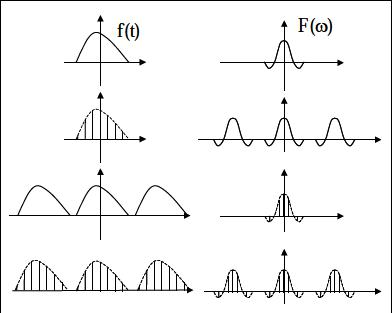## 零、全局符号意义说明

$N$：采样点数目

$\Delta_t$：时域采样间隔

$T_0=N\cdot \Delta_t$：时域采样长度

$W_0=\frac{2\pi}{\Delta_t}$：频域采样长度

$\Delta_\omega=\frac{W_0}{N}=\frac{2\pi}{T_0}$：频域采样间隔

## 一、傅立叶变换（单变量）

(需要说明的是，傅立叶变换有好几种定义方式，本文讨论以上这种形式，但本文中的思适用于所有的形式）

\begin{equation*} X(k)=\text{DFS}(x(n))=\sum_{n=0}^{N-1}x(n)\text{e}^{-\text{i}\frac{2\pi}{N}nk} \end{equation*}

\begin{equation} x(n)=\text{IDFS}(X(k))=\frac{1}{N}\sum_{k=0}^{N-1}X(k)\text{e}^{\text{i}\frac{2\pi}{N}nk} \end{equation}

## 三、常见函数的傅立叶变换对

1、rect函数： $% \frac{T_{0}}{2}\\{1} & -\frac{T_0}{2}\leq t\leq \frac{T_0}{2}\end{cases} %]]>$

2、$\delta函数$（单位冲击函数）：

3、冲击串函数：

## 四、卷积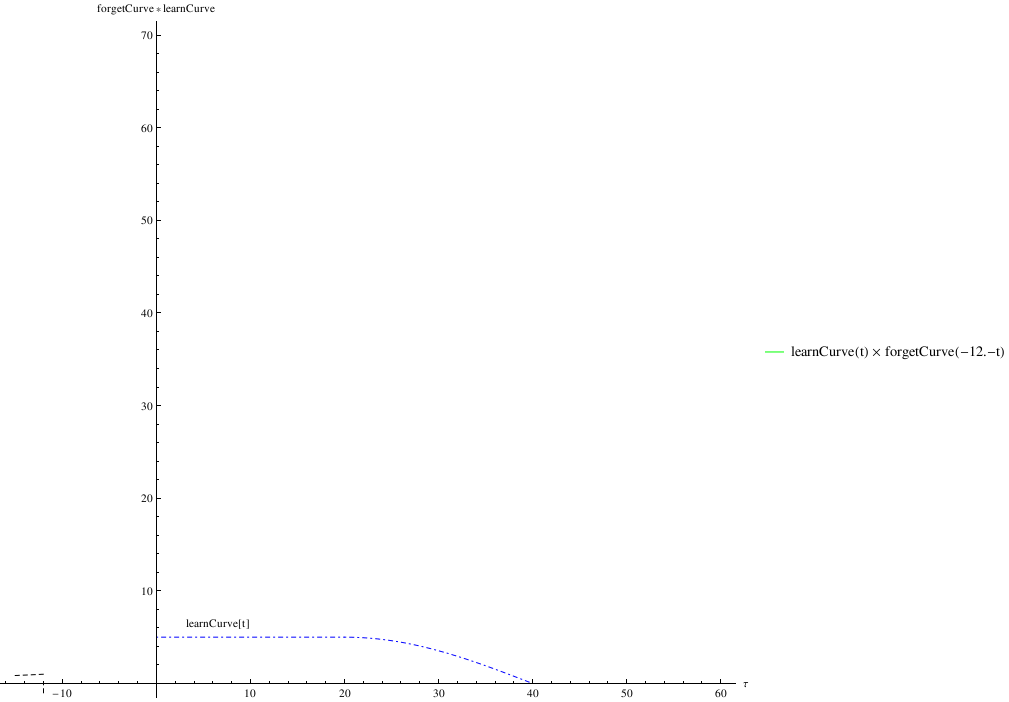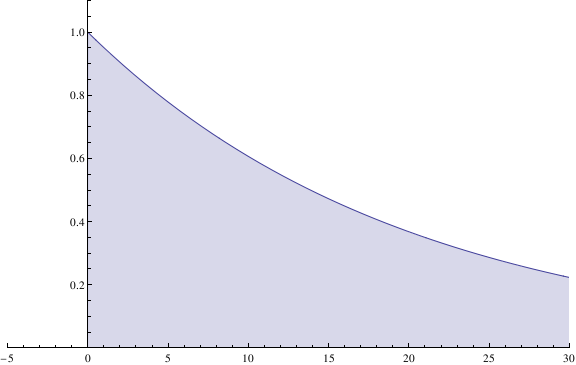$\delta$函数的卷积：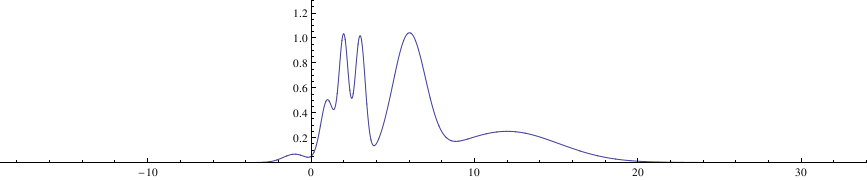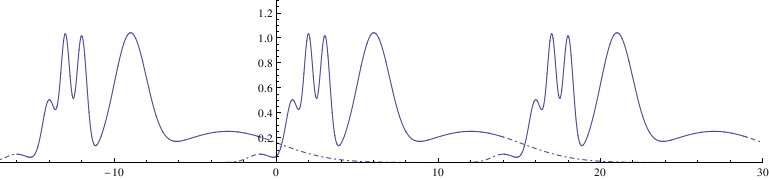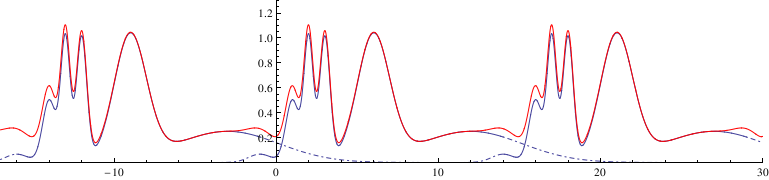## 五、实例

(说明，由于频谱值一般为复数，下面的频谱图Y轴均为$$\text{Abs}(F(\omega)))$$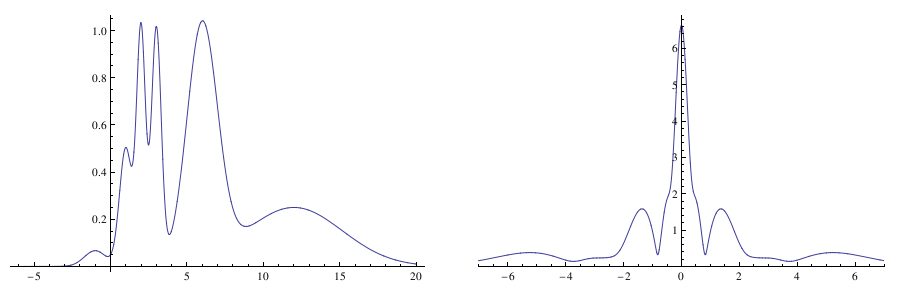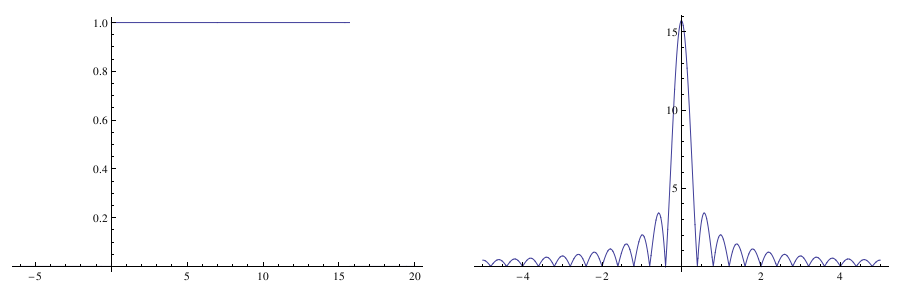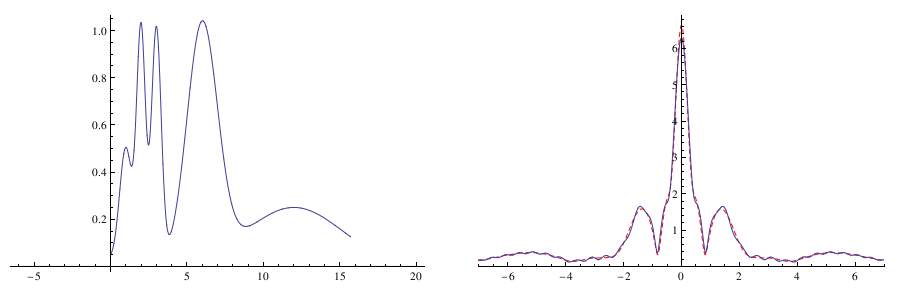(下文中令$$f(t)\cdot{timeWindow(t,T_0)}=f_1(t)$$，$$F(\omega) * (\frac{1}{2\pi}\cdot ItimeWindow(\omega))=F_1(\omega))$$

“等等….”，你会说：“我的采样明明是在一个时间序列上的每个点的一个数值啊，不是一串奇怪的$\delta$函数啊？”

“额…这是个好问题，不过先..别管他吧，我们就看看我们这样做能得到什么吧…”

“好吧….”(下文中令$$f_1(t)\cdot \delta_{\Delta_t}(t)=f_2(t),F_1(\omega) * \frac{1}{\Delta_t}\delta_{W_0}(w)=F_2(\omega))$$

A：“哈哈，加窗、采样后我们进行傅立叶变换的到的频谱，除了稍微有点失真、变成了一个奇怪的周期函数、还有乘以了一个奇怪的$\frac{1}{\Delta_t}$系数以外，和上帝模式的形状长得差不多么，啦啦啦～～”

B：“咳咳…好了，不管你这是什么乱七八糟的东西，赶紧给我频谱数据，老板还等着我的结果呢，你这个数据好像还得乘以$\Delta_t$吧，不然和上帝模式的差好多倍呢”

A：“现在这个有点奇怪的频谱结果如果直接逆变换回去，会得到一个冲击函数序列$f_2(t)$，每个点的冲击函数和那个点你的采样值倍的$\delta$函数一样，并不是你原来的采样序列$f(t_k),k=0,1,2...N-1，t_k=k\cdot \Delta_t$，恩，是有点奇怪，不管了，就把数据给你吧，不过….愚蠢的人类，这是一个无限长的周期频谱啊，你只能取其中的一段”

B：“既然这个一个周期函数，我就取它的一个周期吧，我要$(0,W_0)$这段的数据”

A：“恩，不错，你相当与在频域上加了一个长度为$W_0$的窗，我们令你这个加过窗的频谱为

B：“啥?…..那我们就先看看频域中timeWindow窗逆傅里叶变换以后的形式ItimeWindow吧..如图”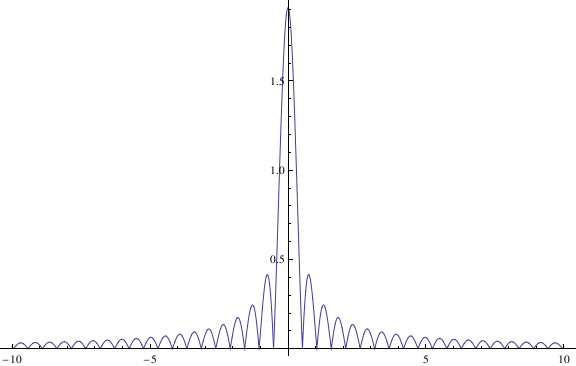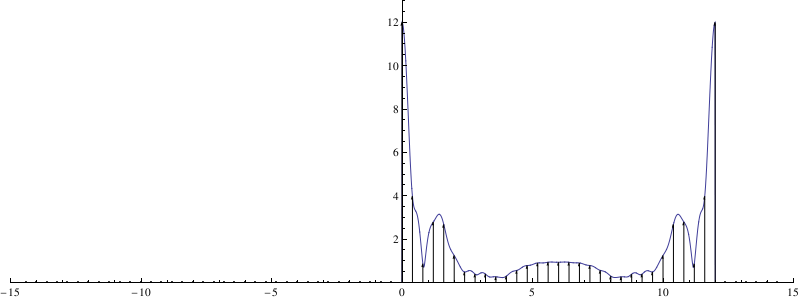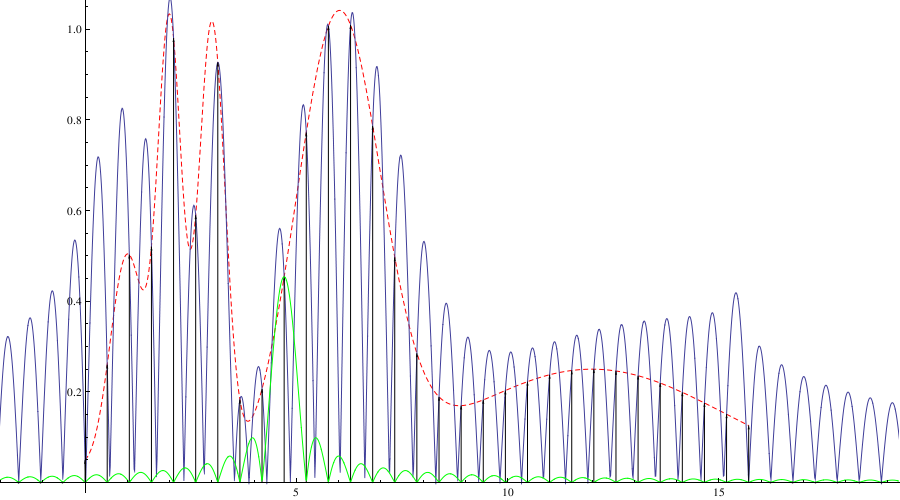$$f_{20}(t)$$最前面的系数为了使其形状和$$f_2(t)$$比较容易比较，而这个傅里叶变换对的真正形式应该如下

B：“好了，我们这一对傅里叶变换总算稍微正常了一点，两边长的都像是正常的函数了，不过$f_3(t)$比我们理想中的结果还多了个$\frac{W_0}{2\pi}$系数，我们是不是考虑把右边的这个频谱再乘个$\frac{2\pi}{W_0}$后再给我？正如上图中的处理一样?”

A：“别急，你不是要数据么，右边的有限区间的连续函数还是没法给你，我们还得采样。”

B：“额，既然也要采样，那么就和之前T的采样一样多得了，也取N个值，采样区间就是$\Delta_\omega=\frac{W_0}{N}$吧”

A：“好的，我们给频域乘以一个单位冲击串函数$\delta_{\Delta_\omega}(w)$

B：“什么？好不容易两端都是正常的函数了，你又把右边弄成了奇怪的冲击串？”

A：“好问题+1….我们不管他，变过去看看什么样再说吧….”

B：“好….吧….别给我说你科研都是这么搞的”

A：“（躺枪….）”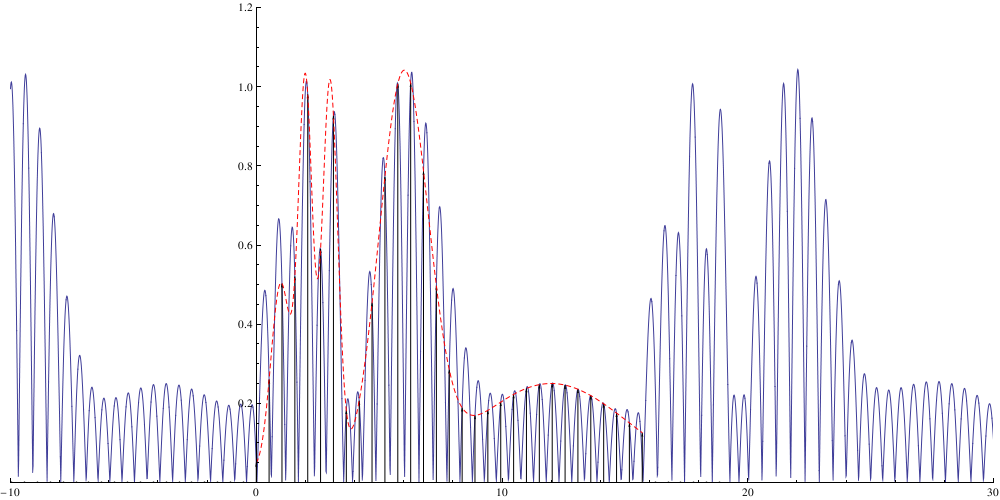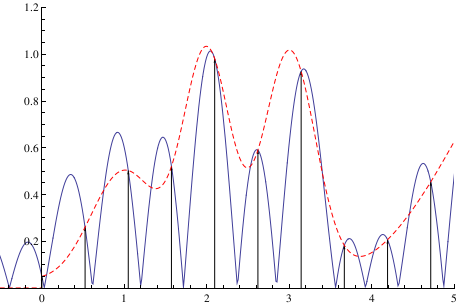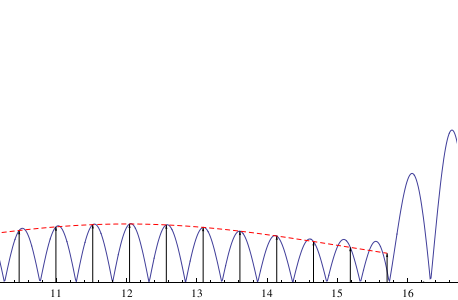A：“大功告成，你看我右边的频谱$F_4(\omega)$终于可以逆变换得到和上帝模式长的一模一样的函数了~~额，不对，是在你的采样点处和上帝模式长的一模一样，但在其他点处跟上帝模式差别就大了…..还有，我们的图是$F_4(\omega)$逆变换过去后又除以$\frac{N}{2\pi}$得到的，恩，你怎么看？”

B：“尼玛我要数据！我要频谱！你这左边又变成了一个奇怪的有限长的冲击串，你给我个啥啊?”

A：“…..我们看看$F_4(\omega)$是怎么变回左边的吧

\begin{eqnarray} f_4(t)&=&\frac{1}{2\pi}\int_{-\infty}^{\infty}F_4(\omega)\cdot \text{e}^{\text{i}wt}\text{d}\omega\ &=&\frac{1}{2\pi}\sum_{k=0}^{N-1}F_3(k\Delta_\omega)\cdot \text{e}^{\text{i}k\Delta_\omega t}\ &=&\frac{N}{2\pi}f_2(t)\ &t=n\cdot \Delta_t , n=0,1,2…N-1&
\end{eqnarray}

B：“你这半天又是卷积又是加窗，还用什么奇怪的冲击函数去所谓的采样，早告诉我用matlab中的fft和ifft不就得了，你看我扔给他数据就直接出来了和你这折腾半天一样的结果，直接plot，这图长的多漂亮啊，这就是频谱啦？拿数据走人，多谢不送了~（闪人）”

A：“喂，你到底弄清楚了你得到的是什么东西了么？人呢？…”

A：“算了，自己总结个吧….一般搞实验的除了自己谁都得相信你的结果，我还是自己再检查一下吧”